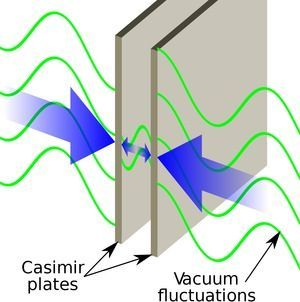BlogFrom the fictional universe of Stargate Atlantis and Marvel Comic’s Realm of Kings to NASA’s Eagleworks Propulsion laboratory, zero-point energy, also known as vacuum energy, is touted as a potentially limitless and ubiquitous source of energy, if one can only find the means to harness it.  Zero-point energy can be formulated in a few different ways, but in its most basic form, it is the minimal yet non-zero energy of a quantum mechanical system. In quantum field theory, zero-point energy can be considered by computing the expected energy of the zero photon mode.  In a system with no physical boundaries, the expected energy of the zero photon mode diverges! Yet, if this energy uniformly permeates all of space-time, it is not directly observable.

Conceptual Framework

For pedagogical reasons, we will consider the popular formulation of zero-point energy. The most interesting and relevant framework for zero-point energy can be understood from the quantum field theory for photons and electrons: quantum electrodynamics. Glossing over an exceptional amount of mathematical and conceptual background, the energy of a state in quantum field theory is computed as an expectation of a Hamiltonian„ which describes the energy of the state in terms of operators acting on wavefunctions. The final computation usually requires an integral over the allowed momenta of particles in the state.